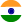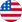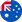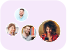24*7 support on WhatsAppChat NowProduct Edition:Illustrated edition
Author: M. P. Hobson
Book Name: General Relativity: An Introduction for Physicists
Subject Name: Science

# General Relativity: An Introduction for Physicists Illustrated edition Solutions

0 out of 5.0
42 reviews44 Students
have requested for homework help from this book

General Relativity: An Introduction for Physicists provides a clear mathematical introduction to Einstein's theory of general relativity. It presents a wide range of applications of the theory, concentrating on its physical consequences. After reviewing the basic concepts, the authors present a clear and intuitive discussion of the mathematical background, including the necessary tools of tensor calculus and differential geometry. These tools are then used to develop the topic of special relativity and to discuss electromagnetism in Minkowski spacetime. Gravitation as spacetime curvature is then introduced and the field equations of general relativity derived. After applying the theory to a wide range of physical situations, the book concludes with a brief discussion of classical field theory and the derivation of general relativity from a variational principle. Written for advanced undergraduate and graduate students, this approachable textbook contains over 300 exercises to illuminate and extend the discussion in the text.

5
21
4
8
3
6
2
5
1
2

0

## Students who viewed this book also checked out